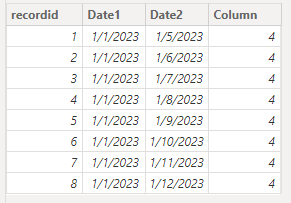cancel
Showing results for
Did you mean:New Member

## DAX - Calculating and Comparing the Difference Between Two Dates, Count Records Based on Results

Hi!

I am still learning the ropes for DAX and need some help. I have used Tableau in the past and know how to do it using their calculated fields, but I can't seem to do it in Power BI.

I am trying to create a measure that does the following: DATEDIFF between two date fields in the same table, counting the number of days between them. Then, count the record if the number of days between the two dates are greater than 7.

This is how I know to do it in Tableau:

Measure 1:
IF (
DATEDIFF('day', [date1], [date2] )
) > 7 then [recordid]
END

Then I could just count this measure.

This is what I have in Power BI (I know this isn't working correctly):

Open More Than 7 Days = IF(
DATEDIFF(
MIN(table_A[date1]),MIN(table_A[date2]),DAY)
> 7, COUNT(table_A[recordid]), 0)

Thank you for your time and any insight you can provide.
1 ACCEPTED SOLUTIONCommunity Support

You can try the following methods.

Column:

``Column = CALCULATE(COUNT(table_A[recordid]),FILTER(table_A,DATEDIFF([date1],[date2],DAY) > 7))``Measure:

``Difference = DATEDIFF( MAX(table_A[date1]),MAX(table_A[date2]),DAY)``
``Open More Than 7 Days = CALCULATE(COUNT(table_A[recordid]),FILTER(ALL(table_A),[Difference]>7))``Is this the result you expect?

Best Regards,

Community Support Team _Charlotte

If this post helps, then please consider Accept it as the solution to help the other members find it more quickly.

2 REPLIES 2Community Support

You can try the following methods.

Column:

``Column = CALCULATE(COUNT(table_A[recordid]),FILTER(table_A,DATEDIFF([date1],[date2],DAY) > 7))``Measure:

``Difference = DATEDIFF( MAX(table_A[date1]),MAX(table_A[date2]),DAY)``
``Open More Than 7 Days = CALCULATE(COUNT(table_A[recordid]),FILTER(ALL(table_A),[Difference]>7))``Is this the result you expect?

Best Regards,

Community Support Team _Charlotte

If this post helps, then please consider Accept it as the solution to help the other members find it more quickly.Super User

@Chris_Dunlap , Create a new column

IF( DATEDIFF(table_A[date1],table_A[date2],DAY) > 7, table_A[recordid], blank() )

or a measure

sumx(table_A, IF( DATEDIFF(table_A[date1],table_A[date2],DAY) > 7, table_A[recordid], blank() ))